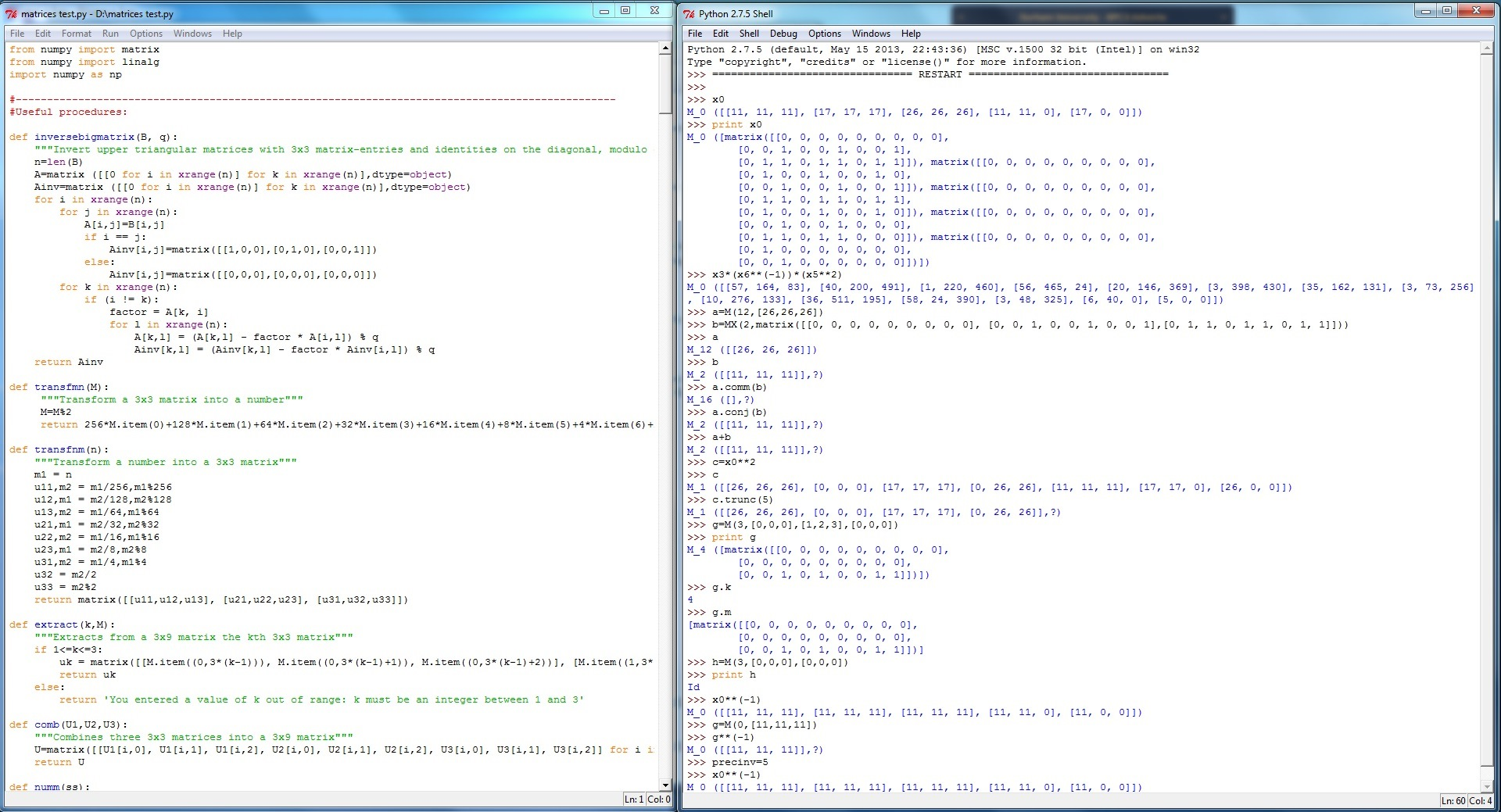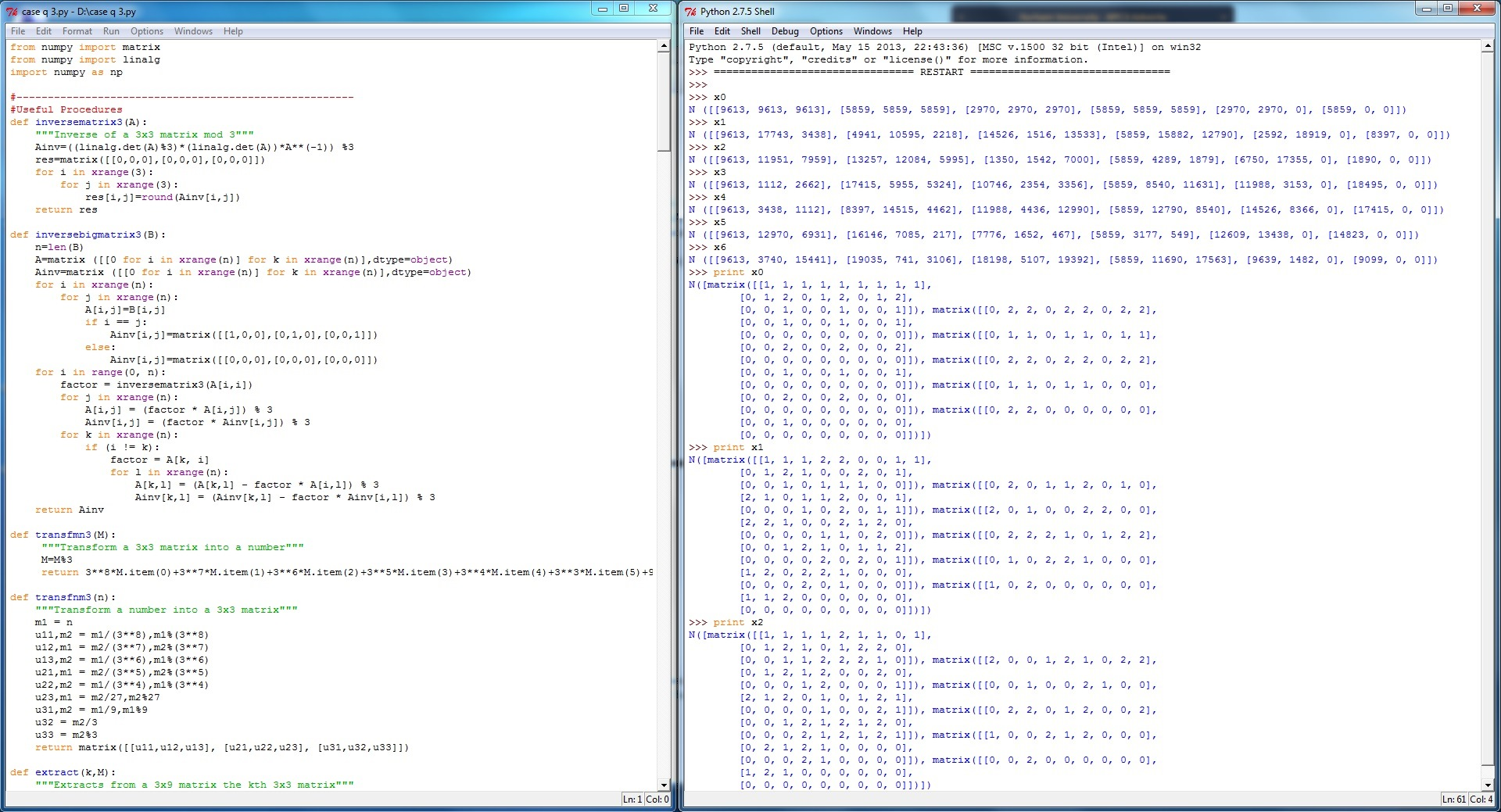## Python programs for matrix computations of groups (F. Bianchi)

### The software below allows to do matrix computations with representations of the two infinite groups Γ = < x0,...,x6 | xi xi+1 xi+3 = Id > and G = < x0,...,x12 | xi3=xi xi+1 xi+3 = Id > Both groups act transitively on the vertices of Euclidean buildings of type Ã2. (See Cartwright/Mantero/Steger/Zappa, Geom. Dedicata 47(2), 143-166, 1993.)

 Python code to work with a representation of the group Γ by infinite upper triangular Toeplitz matrices.Python code to work with a representation of the group G by infinite upper triangular Toeplitz matrices.#### Software